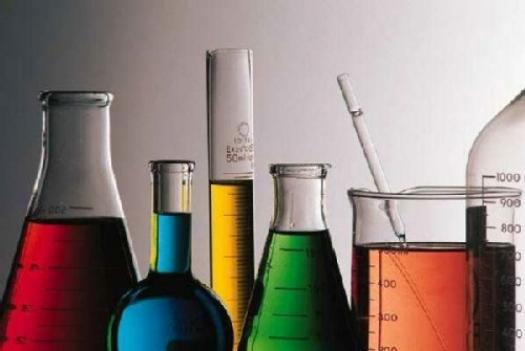# Atomic Structure Exam Quiz

29 Questions | Attempts: 703SettingsElectrons are the smallest of the three particles that make up atoms. Electrons are found in shells or orbitals that surround the nucleus of an atom. Protons and neutrons are found in the nucleus. Test out how much you know about atomic structure by taking the quiz below. All the best!

• 1.
Which answer is the electron configuration of Vanadium, which has an atomic number of 23?
• A.

1s2 2s2 2p6 3s1 3p6 4s1 3d6

• B.

1s2 2s2 2p6 3s2 3p6 4s2 3d7

• C.

1s2 2s2 2p6 3s6 3p6 4s2 3d6

• D.

1s2 2s2 2p6 3s2 3p6 4s2 3d3

• E.

1s2 2s2 2p6 3s2 3p6 4s2 4p3

• 2.
Isotopes have different amounts of...
• A.

Electrons

• B.

Protons

• C.

Neutrons

• D.

Both A & B.

• E.

Both B & C

• 3.
Ions contain different amounts of ...
• A.

Electrons

• B.

Protons

• C.

Neutrons

• D.

Both A & B

• E.

Both A & C

• 4.
Which element is four times the mass of carbon?
• A.

Cr

• B.

Ti

• C.

Cd

• D.

Cl

• E.

Mn

• 5.
Which element has 14 neutrons?
• A.

N

• B.

Br

• C.

Si

• D.

Al

• E.

Both C & D

• 6.
Atomic mass =
• A.

Number of protons

• B.

Number of protons + electrons

• C.

Number of neutrons + electrons

• D.

Number of neutrons + protons

• E.

Number of protons, electrons, & neutrons.

• 7.
Raiderium (Cv) has three naturally occurring isotopes.  Raiderium is 74.655% 44Cv, which has an atomic mass of 43.064 amu, 24.958% 46Cv, which has a mass of 46.125 amu, and 0.387% 48Cv, which has an atomic mass of 47.982 amu.  Please calculate the average atomic mass of Raiderium.
• 8.
How many electrons are in 23Na?
• 9.
If you have an element with 46 protons and 44 electrons, the net charge of the element is ...
• 10.
If you have an element with 86 electrons, 125 neutrons, 82 protons, what is the ionic charge of the atom.
• 11.
What type of particles did Rutherford use in his experiment?
• 12.
Which subatomic particle has no charge?
• 13.
Which electron orbital holds 14 electrons.
• 14.
How many electrons does Mg+2 have?
• 15.
What electron orbital do all the noble gases fill up to be so stable.
• 16.
The atomic number = the number of
• 17.
What units are used to weigh atoms?
• A.

Amu

• B.

G

• C.

Mg

• D.

Kg

• E.

Gelliards

• 18.
A single proton has what electrical charge?
• A.

No charge

• B.

positive charge

• C.

Both positive and negative

• D.

Negative charge

• E.

Either a positive or negative charge

• 19.
Which particles have approximately the same size and mass as each other?
• A.

Neutrons and electrons

• B.

Electrons and protons

• C.

Protons and neutrons

• D.

None - they are all very different in size and mass

• E.

They all have the same mass

• 20.
If an oxygen atom was expanded to the size of a sugar cube, how much would it weigh?
• 21.
According to atomic theory, electrons are usually found:
• A.

In the atomic nucleus.

• B.

outside the nucleus, yet very near it because they are attracted to the protons.

• C.

Everywhere, there is no place it cannot be.

• D.

Either in the nucleus or around it - electrons are readily found anywhere in an atom

• E.

Outside the nucleus and often far from it - most of an atom's volume is its electron cloud.

• 22.
The element 3216S2- contains:
• A.

18 protons and 16 neutrons

• B.

16 protons and 16 electrons

• C.

16 protons and 18 neutrons

• D.

16 protons and 18 electrons

• E.

18 electrons and 32 neutrons

• 23.
Alpha particles have a charge of ...
• 24.
Beta particles have a charge of ...
• 25.
Which radioactive particle is most dangerous to humans?

## Related TopicsBack to top
×

Wait!
Here's an interesting quiz for you.